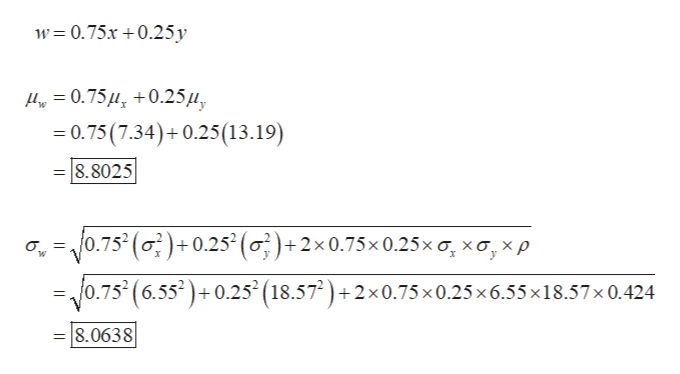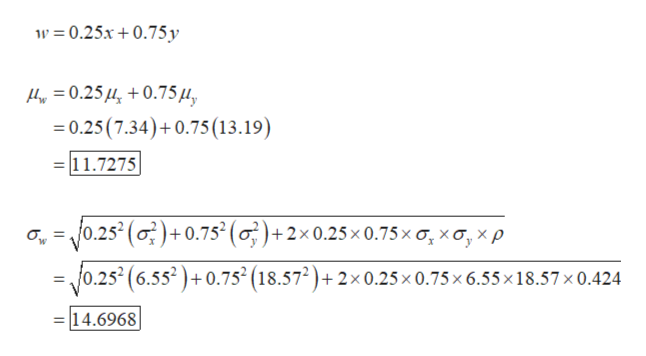# 2. Previously, you studied linear combinations of independent random variables. What happens if the variables are not independent? A lot of mathematics can be used to prove the following: Let x and y be random variables with means μx and μy, variances σ2x and σ2y, and population correlation coefficient ρ (the Greek letter rho). Let a and b be any constants and let w = ax + by for the following formula.μw = aμx + bμyσ2w = a2σ2x + b2σ2y + 2abσxσyρIn this formula, r is the population correlation coefficient, theoretically computed using the population of all (x, y) data pairs. The expression σxσyρ is called the covariance of x and y. If x and y are independent, then ρ = 0 and the formula for σ2w reduces to the appropriate formula for independent variables. In most real-world applications the population parameters are not known, so we use sample estimates with the understanding that our conclusions are also estimates.Do you have to be rich to invest in bonds and real estate? No, mutual fund shares are available to you even if you aren't rich. Let x represent annual percentage return (after expenses) on the Vanguard Total Bond Index Fund, and let y represent annual percentage return on the Fidelity Real Estate Investment Fund. Over a long period of time, we have the following population estimates.μx ≈ 7.34,      σx ≈ 6.55,      μy ≈ 13.19,      σy ≈ 18.57,      ρ ≈ 0.424(a) Do you think the variables x and y are independent? Explain your answer.Yes. Interest rates probably has no effect on the investment returns.No. Interest rates probably has no effect on the investment returns.  No. Interest rate probably affects both investment returns.Yes. Interest rate probably affects both investment returns.(b) Suppose you decide to put 75% of your investment in bonds and 25% in real estate. This means you will use a weighted average w = 0.75x + 0.25y. Estimate your expected percentage return μw and risk σw.μw =  σw =  (c) Repeat part (b) if w = 0.25x + 0.75y.μw =  σw =  (d) Compare your results in parts (b) and (c). Which investment has the higher expected return? Which has the greater risk as measured by σw?w = 0.75x + 0.25y produces higher return with lower risk as measured by σw.w = 0.25x + 0.75y produces higher return with lower risk as measured by σw.w = 0.25x + 0.75y produces higher return with greater risk as measured by σw.Both investments produce the same return with the same risk as measured by σw.w = 0.75x + 0.25y produces higher return with greater risk as measured by σw.

Question

2. Previously, you studied linear combinations of independent random variables. What happens if the variables are not independent? A lot of mathematics can be used to prove the following: Let x and y be random variables with means μx and μy, variances σ2x and σ2y, and population correlation coefficient ρ (the Greek letter rho). Let a and b be any constants and let w = ax + by for the following formula.

μw = aμx + bμy
σ2w = a2σ2x + b2σ2y + 2abσxσyρ

In this formula, r is the population correlation coefficient, theoretically computed using the population of all (xy) data pairs. The expression σxσyρ is called the covariance of x and y. If x and y are independent, then ρ = 0 and the formula for σ2w reduces to the appropriate formula for independent variables. In most real-world applications the population parameters are not known, so we use sample estimates with the understanding that our conclusions are also estimates.

Do you have to be rich to invest in bonds and real estate? No, mutual fund shares are available to you even if you aren't rich. Let x represent annual percentage return (after expenses) on the Vanguard Total Bond Index Fund, and let y represent annual percentage return on the Fidelity Real Estate Investment Fund. Over a long period of time, we have the following population estimates.

μx ≈ 7.34,      σx ≈ 6.55,      μy ≈ 13.19,      σy ≈ 18.57,      ρ ≈ 0.424

(a) Do you think the variables x and y are independent? Explain your answer.
Yes. Interest rates probably has no effect on the investment returns.
No. Interest rates probably has no effect on the investment returns.
No. Interest rate probably affects both investment returns.
Yes. Interest rate probably affects both investment returns.

(b) Suppose you decide to put 75% of your investment in bonds and 25% in real estate. This means you will use a weighted average w = 0.75x + 0.25y. Estimate your expected percentage return μw and risk σw.
μw =
σw =

(c) Repeat part (b) if w = 0.25x + 0.75y.
μw =
σw =
(d) Compare your results in parts (b) and (c). Which investment has the higher expected return? Which has the greater risk as measured by σw?
w = 0.75x + 0.25y produces higher return with lower risk as measured by σw.
w = 0.25x + 0.75y produces higher return with lower risk as measured by σw.
w = 0.25x + 0.75y produces higher return with greater risk as measured by σw.
Both investments produce the same return with the same risk as measured by σw.
w = 0.75x + 0.25y produces higher return with greater risk as measured by σw.
check_circleExpert Solution
Step 1

Consider the provided information:

μx ≈ 7.34,      σx ≈ 6.55,      μy ≈ 13.19,      σy ≈ 18.57,      ρ ≈ 0.424

(a)

Here, the population correlation coefficient is not zero and it is positive.

So, the variables x and y are not independent. Therefore, the correct option is, ‘No. Interest rate probably affects both investment returns’.

Step 2

(b)

The required values are calculated below:help_outlineImage Transcriptionclosew%3D0.75x + 0.25у д, 3 0.75 и, +0.25и, =0.75 (7.34)+0.25(13.19) 8.8025 yo,75 (о.75 (6.55')+0.25' (18.57) +2х0.75х0.25x6.55х18.57x0.424 0.75° () +0.25°(о)+2x0.75х0.25ха, ха, хр w 8.0638 fullscreen
Step 3

(c)

The required values are ...help_outlineImage Transcriptionclosew 0.25x0.75y 0.250.75 u, 0.25 (7.34) 0.75 (13.19) 11.7275 = f0.25° (o) +0.75° (oF)+ +2x 0.25 x 0.75 x o, xO, x p 0.252(6.552)+0.752 (18.572)+2x 0.25x 0.75 x 6.55 x 18.57 x 0.424 14.6968 fullscreen

### Want to see the full answer?

See Solution

#### Want to see this answer and more?

Solutions are written by subject experts who are available 24/7. Questions are typically answered within 1 hour*

See Solution
*Response times may vary by subject and question
Tagged in

### Other